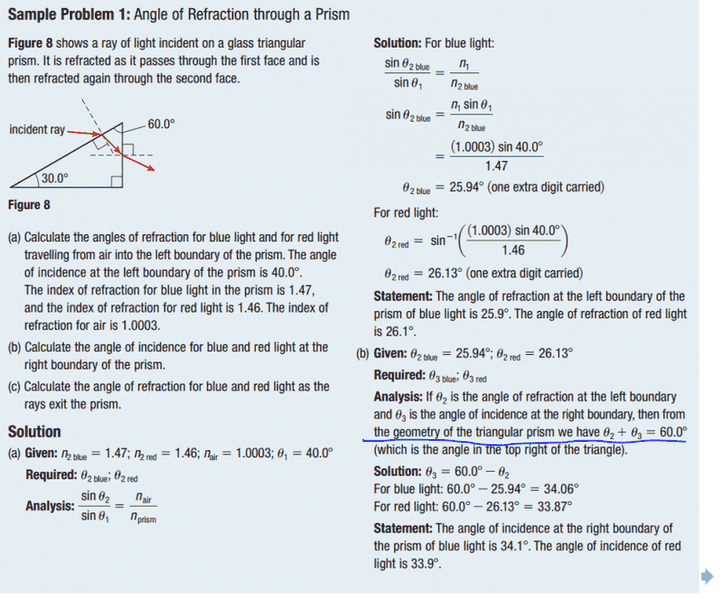# Refraction and PrismsThat is a page from my textbook. The book mentions that the angle of incidence on the right side when added with the angle of the refraction equals 60 degrees. Why is that?

## Answers and Replies

mfb
Mentor
Consider the triangle formed by the light ray in the prism and the two prism borders. The sum of the interior angles is 180°, and one angle is 60°. Everything else follows from geometry with the right angles there.

Which triangle? If you mean the one formed by the refracted ray, it is not a right angle triangle.

sophiecentaur
Science Advisor
Gold Member
2020 Award
Another way to look at it. The very top triangle has one angle of 60°. The other two angles are 90- i and 90-r etc. etc.....

mfb
Mentor
Another way to look at it. The very top triangle has one angle of 60°. The other two angles are 90- i and 90-r etc. etc.....
That is the triangle I meant.
And the sum of those three angles is 180 degrees.